# Evolution of Neuro-Controllers for Robots' Alignment using Local Communication

by Álvaro Gutiérrez Martín, Elio Tuci and Alexandre Campo
July 2008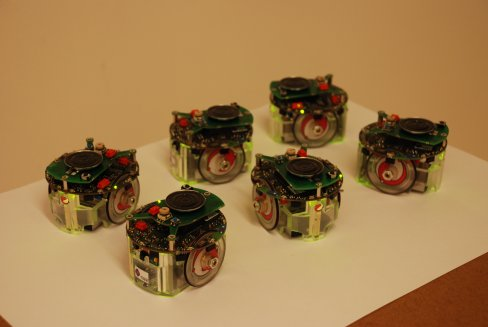## Polarisation data of Real Experiments:

We tested the best genotype obtained by artificial evolution in groups of 3, 4, and 6 physical robots across 30 repeated experiments. We use a specific measure of polarisation to calculate the degree of alignment of all the robots. Polarisation P(G) of a group of robots G is defined using the angular nearest neighbour: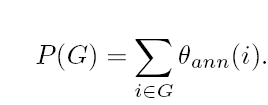where θann(i) is the relative orientation of the angular nearest neighbour of the robot i. If all robots are aligned, then P(G) = 0. Conversely, if headings are evenly distributed, P(G) = 2π. If headings are random, i.e. drawn from a uniform distribution, then P(G) = π in average.

All the data collected on real experiments are in Data Section.

## Spatial arrangements of the robots in each post-evaluation test

In this section we show the spatial arrangements of the e-pucks for the 3, 4 and 6 and robot experiments.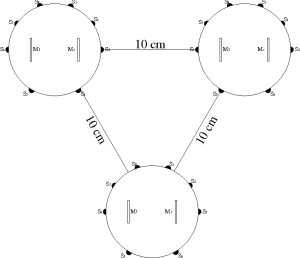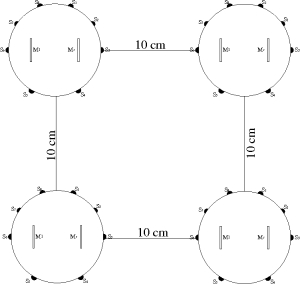(a) (b)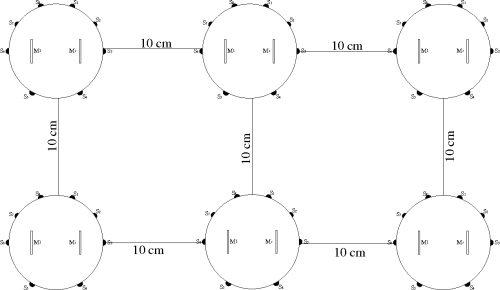(c)

Figure 1: Pictures showing the spatial configurations the robots are forming in the (a) three robots test; (b) four robots tests; (c) six robots tests; In these pictures the robots are depicted facing the same direction. However, the reader should bear in mind that, at the beginning of a post-evaluation trial, the robots are facing randomly chosen orientation.

## Real Experiments Movies

All movies recorded can be found at Movies Section.